# Dictionary Learning for Denoising¶

Important: Please read the installation page for details about how to install the toolboxes. $\newcommand{\dotp}{\langle #1, #2 \rangle}$ $\newcommand{\enscond}{\lbrace #1, #2 \rbrace}$ $\newcommand{\pd}{ \frac{ \partial #1}{\partial #2} }$ $\newcommand{\umin}{\underset{#1}{\min}\;}$ $\newcommand{\umax}{\underset{#1}{\max}\;}$ $\newcommand{\umin}{\underset{#1}{\min}\;}$ $\newcommand{\uargmin}{\underset{#1}{argmin}\;}$ $\newcommand{\norm}{\|#1\|}$ $\newcommand{\abs}{\left|#1\right|}$ $\newcommand{\choice}{ \left\{ \begin{array}{l} #1 \end{array} \right. }$ $\newcommand{\pa}{\left(#1\right)}$ $\newcommand{\diag}{{diag}\left( #1 \right)}$ $\newcommand{\qandq}{\quad\text{and}\quad}$ $\newcommand{\qwhereq}{\quad\text{where}\quad}$ $\newcommand{\qifq}{ \quad \text{if} \quad }$ $\newcommand{\qarrq}{ \quad \Longrightarrow \quad }$ $\newcommand{\ZZ}{\mathbb{Z}}$ $\newcommand{\CC}{\mathbb{C}}$ $\newcommand{\RR}{\mathbb{R}}$ $\newcommand{\EE}{\mathbb{E}}$ $\newcommand{\Zz}{\mathcal{Z}}$ $\newcommand{\Ww}{\mathcal{W}}$ $\newcommand{\Vv}{\mathcal{V}}$ $\newcommand{\Nn}{\mathcal{N}}$ $\newcommand{\NN}{\mathcal{N}}$ $\newcommand{\Hh}{\mathcal{H}}$ $\newcommand{\Bb}{\mathcal{B}}$ $\newcommand{\Ee}{\mathcal{E}}$ $\newcommand{\Cc}{\mathcal{C}}$ $\newcommand{\Gg}{\mathcal{G}}$ $\newcommand{\Ss}{\mathcal{S}}$ $\newcommand{\Pp}{\mathcal{P}}$ $\newcommand{\Ff}{\mathcal{F}}$ $\newcommand{\Xx}{\mathcal{X}}$ $\newcommand{\Mm}{\mathcal{M}}$ $\newcommand{\Ii}{\mathcal{I}}$ $\newcommand{\Dd}{\mathcal{D}}$ $\newcommand{\Ll}{\mathcal{L}}$ $\newcommand{\Tt}{\mathcal{T}}$ $\newcommand{\si}{\sigma}$ $\newcommand{\al}{\alpha}$ $\newcommand{\la}{\lambda}$ $\newcommand{\ga}{\gamma}$ $\newcommand{\Ga}{\Gamma}$ $\newcommand{\La}{\Lambda}$ $\newcommand{\si}{\sigma}$ $\newcommand{\Si}{\Sigma}$ $\newcommand{\be}{\beta}$ $\newcommand{\de}{\delta}$ $\newcommand{\De}{\Delta}$ $\newcommand{\phi}{\varphi}$ $\newcommand{\th}{\theta}$ $\newcommand{\om}{\omega}$ $\newcommand{\Om}{\Omega}$

This tour follows the Dictionary Learning numerical tour. It uses a learned dictionary to perform image denoising.

In :
addpath('toolbox_signal')


## Learning the Dictionary¶

We aim at applying the dictionary learning method to denoising. We thus consider a noisy image obtained by adding Gaussian noise to a clean, unknown image $f_0 \in \RR^N$ where $N=n_0 \times n_0$.

Noise level $\sigma$.

In :
sigma = .06;


Size of the image.

In :
n0 = 256;


In :
name = 'barb';


Display $f_0$.

In :
clf;
imageplot(f0);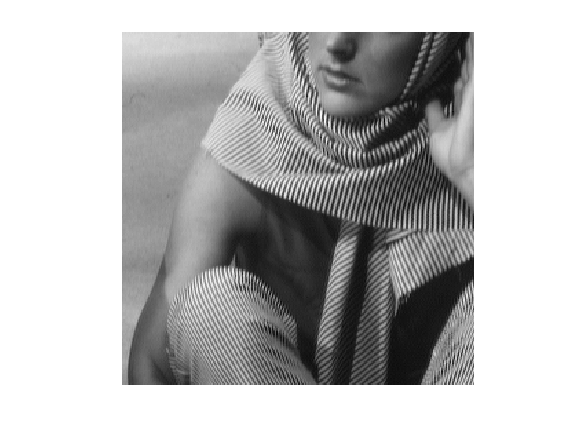Noisy observations $f=f_0+w$ where $w$ is a realization of $\Nn(0,\si^2\text{Id}_N)$.

In :
f = f0 + sigma*randn(n0);


Display $f$.

In :
clf;
imageplot(f);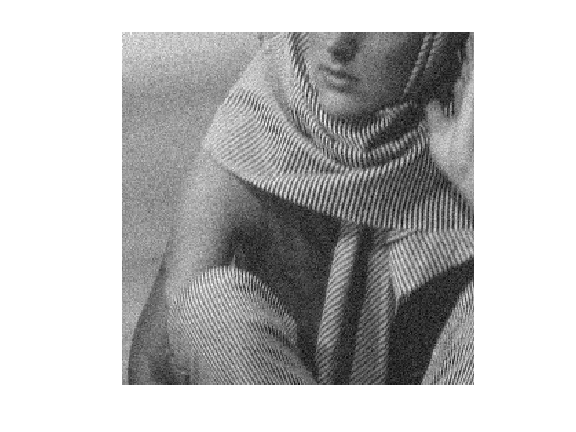Perform the numerical tour on Dictionary Learning to obtain a dictionary $D \in \RR^{n \times p}$

In :
if not(exist('D'))
sparsity_4_dictionary_learning;
end

[Warning: Name is nonexistent or not a directory: ../toolbox_signal]
[> In path at 110
In perform_toolbox_installation>@(p)path(p,path) at 9
In perform_toolbox_installation at 12
In sparsity_4_dictionary_learning at 6
In pymat_eval at 38
In matlabserver at 27]
[Warning: Name is nonexistent or not a directory: ../toolbox_general]
[> In path at 110
In perform_toolbox_installation>@(p)path(p,path) at 9
In perform_toolbox_installation at 12
In sparsity_4_dictionary_learning at 6
In pymat_eval at 38
In matlabserver at 27]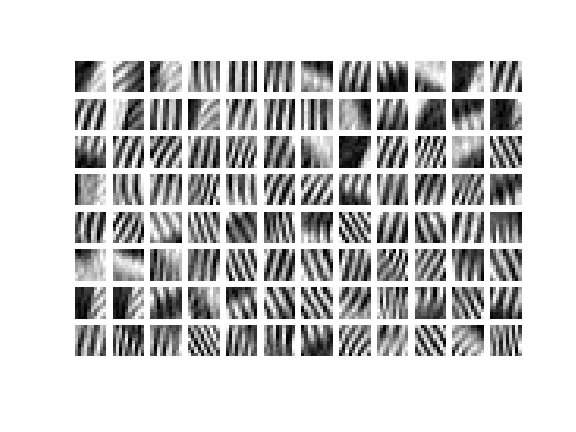Width $w$ of the patches.

In :
w = sqrt(size(D,1));


Number $p$ of atoms.

In :
p = size(D,2);


Dimension $n= w \times w$ of the data to be sparse coded.

In :
n = w*w;


## Denoising by Sparse Coding¶

Here we apply sparse coding in the learned dictionary to the problem of image denoising, as detailed in:

M. Elad and M. Aharon, <http://ieeexplore.ieee.org/xpl/freeabs_all.jsp?arnumber=4011956 Image Denoising Via Sparse and Redundant representations over Learned Dictionaries>, IEEE Trans. on Image Processing, Vol. 15, no. 12, pp. 3736-3745, December 2006.

The method first extract lots of patches from $f$, then perform sparse coding of each patch in $D$, and then average the overlapping patch to obtained the denoised image $f_1$.

We extract a large number $m$ of patches $Y = (y_j)_{j=1}^m \in \RR^{n \times m}$ from the noisy image $f$.

To extract the patche, we use $$y_j(t) = f( a_j + t )$$ where $a_j$ is the location of patch indexed by $j$.

In practice, splitting $j=(j_1,j_2)$ as a 2-D index, we use $a_j = (j_1 q,j_2 q)$ where $q>0$ is an overlap factor ( so that setting $q=w$ implies no overlap). Overlap is important to obtain good denoising performance (reduced blocking artifact).

In :
q = 2;


Define regularly space positions for the extraction of patches.

In :
[y,x] = meshgrid(1:q:n0-w/2, 1:q:n0-w/2);
m = size(x(:),1);
Xp = repmat(dX,[1 1 m]) + repmat( reshape(x(:),[1 1 m]), [w w 1]);
Yp = repmat(dY,[1 1 m]) + repmat( reshape(y(:),[1 1 m]), [w w 1]);


Ensure boundary conditions (reflexion).

In :
Xp(Xp>n0) = 2*n0-Xp(Xp>n0);
Yp(Yp>n0) = 2*n0-Yp(Yp>n0);


Extract the $m$ patches $Y$.

In :
Y = f(Xp+(Yp-1)*n0);
Y = reshape(Y, [n, m]);


Save the mean $\theta_j$ of each patch appart, and remove it.

In :
theta = mean(Y);
Y = Y - repmat( theta, [n 1] );


Denoising of the patches is obtained by performing a sparse coding of each patch $y_j$ in $D$ $$\umin{\norm{D x_j - y_j} \leq \epsilon} \norm{x_j}_1.$$

The value of $\epsilon>0$ is set proportionaly to the noise level $\sqrt{n}\sigma$ that contaminates each patch. We denote $\rho \approx 1$ the proportionality factor, that is a parameter of the method.

In :
rho = .95;
epsilon = rho*sqrt(n)*sigma;


The sparse coding problem can written equivalently in a way that is easier to deal using proximal splitting schemes. We introduce an auxiliary variable $u=D x \in \RR^n$ as follow $$\umin{ z=(x,u) \in \RR^p \times \RR^n } F(z) + G(z)$$ where for $z=(x,u)$, one defines $$F(x,u) = \norm{x}_1 + \iota_{B_\epsilon(y)}(u) \qwhereq B_\epsilon(y) = \enscond{u}{ \norm{u-y} \leq \epsilon }$$ and $$G(x,u) = \iota_{\Cc}(x,u) \qwhereq \Cc = \enscond{(x,u)}{ u=D x }.$$

Here we included the constraints using characteristic functions $$\iota_{A}(z) = \choice{ 0 \qifq z \in A, \\ +\infty \quad \text{otherwise}. }$$

## Douglas-Rachford Algorithm¶

To minimize the sparse coding problem, we make use of a proximal splitting scheme to minimize an energy of the form $F(z)+G(z)$. These schemes are adapted to solve structured non-smooth optimization problem.

They basically replace the traditional gradient-descent step (that is not available because neither $F$ nor $G$ are smooth functionals) by proximal mappings, defined as $$\text{Prox}_{\gamma F}(z) = \uargmin{y} \frac{1}{2}\norm{z-y}^2 + \ga F(y)$$ (the same definition applies also for $G$).

The Douglas-Rachford (DR) algorithm is an iterative scheme to minimize functionals of the form $$\umin{z} F(z) + G(z)$$ where $F$ and $G$ are convex functions for which one is able to comptue the proximal mappings $\text{Prox}_{\gamma F}$ and $\text{Prox}_{\gamma G}$.

The important point is that $F$ and $G$ do not need to be smooth. One onely needs then to be "proximable".

A DR iteration reads $$\tilde z_{k+1} = \pa{1-\frac{\mu}{2}} \tilde z_k + \frac{\mu}{2} \text{rPox}_{\gamma G}( \text{rProx}_{\gamma F}(\tilde z_k) ) \qandq z_{k+1} = \text{Prox}_{\gamma F}(\tilde z_{k+1},)$$

We have use the following shortcuts: $$\text{rProx}_{\gamma F}(z) = 2\text{Prox}_{\gamma F}(z)-z$$

It is of course possible to inter-change the roles of $F$ and $G$, which defines another set of iterations.

One can show that for any value of $\gamma>0$, any $0 < \mu < 2$, and any $\tilde z_0$, $z_k \rightarrow z^\star$ which is a minimizer of $F+G$.

Please note that it is actually $z_k$ that converges, and not $\tilde z_k$.

Proximal Splitting Methods in Signal Processing, Patrick L. Combettes and Jean-Christophe Pesquet, in: Fixed-Point Algorithms for Inverse Problems in Science and Engineering, New York: Springer-Verlag, 2010.

## Douglas-Rachford for Sparse Coding¶

In the special case of the constrained sparse coding problem, the proximal mapping of $G$ is the orthogonal projection on the convex set $\Cc$: $$(\tilde x, \tilde u) = \text{Prox}_{\ga G}(x,u) = \text{Proj}_\Cc(x,u).$$

It can be computed by solving a linear system of equations since $$\tilde u = D \tilde x \qwhereq \tilde x = (\text{Id} + D^* D)^{-1}(f + D^* u).$$

Define Proj$_\Cc$, by pre-compuring the inverse of $\text{Id} + D^* D$.

In :
U = (eye(p) + D'*D)^(-1);
Replicate = @(z)deal(z, D*z);
ProjC = @(x,u)Replicate( U*( x + D'*u ) );


One has $\text{Prox}_{\ga G} = \text{Proj}_{\Cc}$, whatever the value of $\ga$.

In :
ProxG = @(f,u,gamma)ProjC(f,u);


Function $F(x,u)$ is actully a separable sum of a function that only depends on $x$ and a function that depends only on $u$: $$F(x,u) = \iota_{B_\epsilon(y)}(u) + \norm{x}_1.$$ The proximal operator of $F$ reads $$\text{Prox}_{\ga F}(x,u) = ( \text{Proj}_{B_\epsilon(y)}(u), \text{Prox}_{\ga \norm{\cdot}_1 }(x) ).$$

In order to speed up the implementation, the DR algorithm will be performed in parallel on all the $x_j$. We thus define $y=Y$ to be the set of all the patches.

In :
y = Y(:,1:m);


Define the projector $$\text{Proj}_{B_\epsilon(y)}(u) = y + (u-y) \max\pa{1 , \frac{\epsilon}{\norm{u-y}} }$$

In :
amplitude = @(a)repmat( sqrt(sum(a.^2,1)), [n 1] );
ProjB = @(u)y + (u-y) .* min(1, epsilon./amplitude(u-y) );


The proximal operator of the $\ell^1$ norm $\norm{\cdot}_1$ is a soft thresholding: $$\text{Prox}_{\ga \norm{\cdot}_1}(x)_i = \max\pa{ 0, \frac{\ga}{\abs{x_i}} } x_i.$$

In :
ProxL1 = @(x,gamma)max(0,1-gamma./max(1e-9, abs(x))) .* x;


Define the proximal operator of $F$.

In :
ProxF = @(x,u,gamma)deal( ProxL1(x,gamma), ProjB(u) );


Set the value of $\mu$ and $\gamma$. You might consider using your own value to speed up the convergence.

In :
mu = 1;
gamma = 1;


Number of iterations.

In :
niter = 800;


Exercise 1

Implement the DR iterative algorithm on |niter| iterations. Keep track of the evolution of the minimized energy $\norm{x}_1$ during the iterations.

In :
exo1()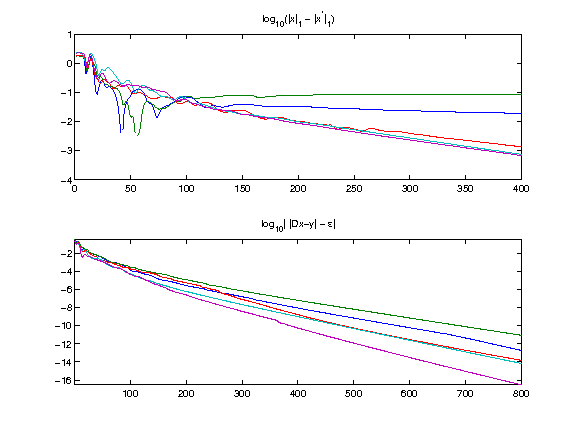In :
%% Insert your code here.


## Patch Averaging¶

Once each $x_j$ is computed, one obtains the denoised patch $\tilde y_j = D x_j$. These denoised patches are then aggregated together to obtained the denoised image: $$f_1(t) = \frac{1}{W_t} \sum_j \tilde y_j(t-a_j)$$ where $W_t$ is the number of patches that overlap at a given pixel location $t$ (note that in this formula, we assumed $\tilde y_j(t-a_j)=0$ when $t-a_j$ falls outside the patch limits).

Approximated patches $Y_1 = (\tilde y_j)_j = D X$.

In :
Y1 = reshape(D*x, [w w m]);


Insert back the mean.

In :
Y1 = Y1 - repmat( mean(mean(Y1)), [w w] );
Y1 = Y1 + reshape(repmat( theta, [n 1] ), [w w m]);


To obtain the denoising, we average the value of the approximated patches $Y_1$ that overlap.

In :
W = zeros(n0,n0);
f1 = zeros(n0,n0);
for i=1:m
x = Xp(:,:,i); y = Yp(:,:,i);
f1(x+(y-1)*n0) = f1(x+(y-1)*n0) + Y1(:,:,i);
W(x+(y-1)*n0) = W(x+(y-1)*n0) + 1;
end
f1 = f1 ./ W;


Display the result.

In :
clf;
imageplot(clamp(f1), ['Denoised, SNR=' num2str(snr(f0,f1),4) 'dB']);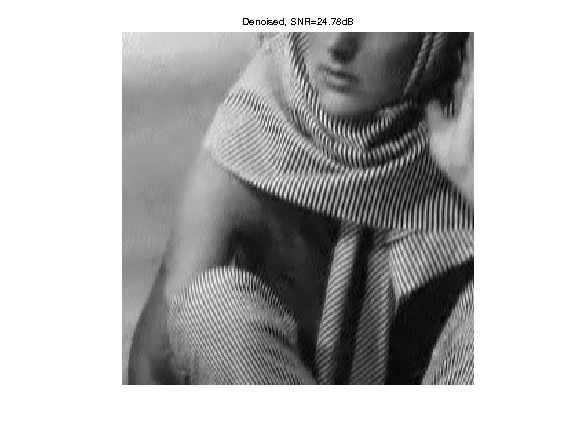Exercise 2

Compare the obtained result with translation invariant wavelet hard thresholding.

In :
exo2()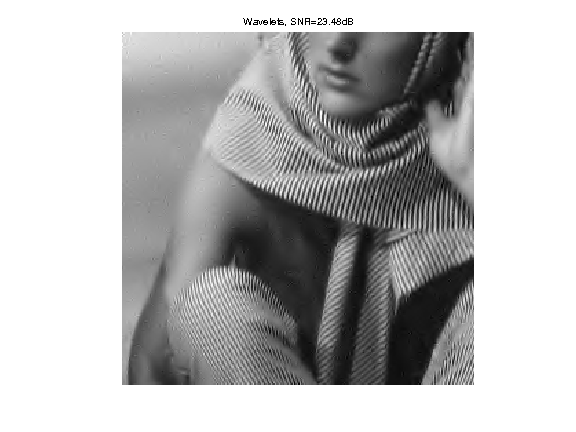In :
%% Insert your code here.


Exercise 3

Study the influence of the parameter $\rho$ on the quality of the denoising. Study the influence of the number $p$ of atoms.

In :
exo3()

In :
%% Insert your code here.